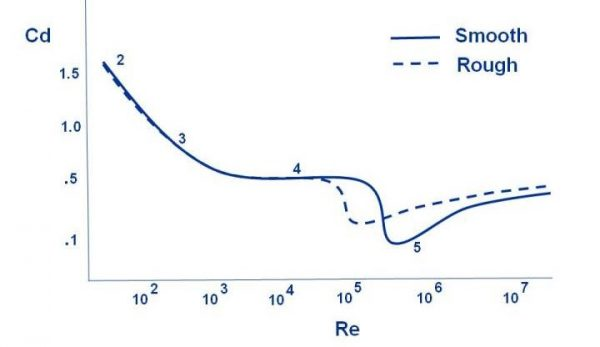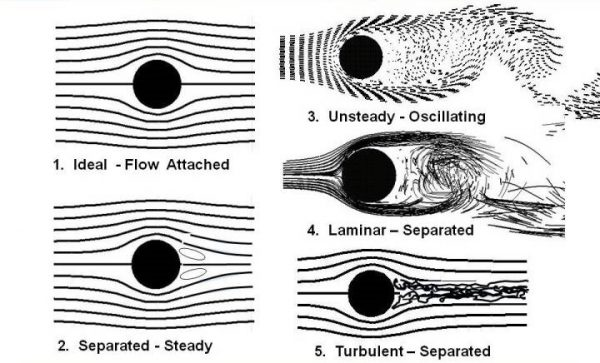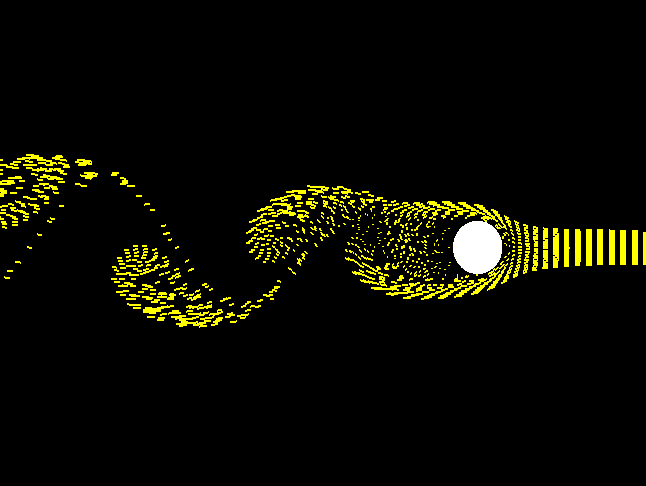# Drag of a SphereThe aerodynamic drag on an object depends on several factors, including the shape, size, inclination, and flow conditions. All of these factors are related to the value of the drag through the drag equation.

### D = Cd * .5 * rho * V^2 * A

Where D is equal to the drag, rho is the air density, V is the velocity, A is a reference area, and Cd is the drag coefficient.

## Drag Coefficient

The drag coefficient is a dimensionless number that characterizes all of the complex factors that affect drag. The drag coefficient is usually determined experimentally using a model in a wind tunnel. In the tunnel, the velocity, density, and size of the model are known. Measuring the drag then determines the value of the drag coefficient as given by the above equation. The drag coefficient and the drag equation can then be used to determine the drag on a similar shaped object at different flow conditions as long as several flow similarity parameters are matched. In particular, Mach number similarity insures that the compressibility effects are correctly modeled, and Reynolds number similarity insures that the viscous effects are correctly modeled. The Reynolds number is the ratio of the inertia forces to the viscous forces and is given by:

### Re = V * rho * l / mu

where l is a reference length, and mu is the viscosity coefficient. For most aerodynamic objects, the drag coefficient has a nearly constant value across a large range of Reynolds numbers.

But for a simple sphere, the value of the drag coefficient varies widely with Reynolds number as shown on the figure at the top of this page. To understand these variations, we are going to look in some detail at the flow past a cylinder. The two dimensional flow past a cylinder is very similar to the three dimensional flow past a sphere, but is a little easier to compute and understand because of the reduced dimensionality. Flow past a cylinder and a sphere goes through a number of transitions with velocity as shown in this figure:In all of the cases presented on this figure, the density, viscosity, and diameter of the ball are the same. The flow velocity is gradually increased from the left to increase the Reynolds number. The drag for each case number is noted on the figure at the top of the page near the drag curve.

## Cases of Flow Past a Cylinder and a Sphere

1. Case 1 shows very slow flow in which we have neglected viscosity entirely. We have an ideal flow with no boundary layer along the surface, completely attached flow (no separation) and no viscous wake downstream of the cylinder. Because the flow is symmetric from upstream to downstream, there is no drag on the cylinder! This strange result is called d’Alembert’s paradox after the early mathematician who studied the problem. Neglecting viscosity simplifies the analysis, but this type of flow does not occur in nature where there is always some small amount of viscosity present in any fluid.
2. Case 2 illustrates what actually occurs for low velocity. A stable pair of vortices are formed on the downwind side of the cylinder. The flow is separated but steady and the vortices generate a high drag on the cylinder or sphere.
3. Case 3 shows the flow as velocity is increased. The downstream vortices become unstable, separate from the body and are alternately shed downstream. The wake is very wide and generates a large amount of drag. The alternate shedding is called the Karman vortex street. Here is a computer animation of the shedding, with the flow going from right to left.This type of flow is periodic, it is unsteady but repeats itself at some time interval. The pressure variation associated with the velocity changes produces a sound called an aeolian tone. This is the sound you hear when the wind blows over high-power wires or past tree limbs in the fall or winter. It is a low frequency (low pitch), haunting tone.

4. Case 4 shows the flow as the velocity is increased even more. The periodic flow breaks down into a chaotic wake. The flow in the boundary layer on the windward side of the cylinder is laminar and orderly and the chaotic wake is initiated as the flow turns onto the leeward side of the cylinder. The wake is not quite as wide as for Case 3, so the drag is slightly less.

5. Case 5 shows the flow at even higher velocity. The boundary layer transitions to chaotic turbulent flow with vortices of many different scales being shed in a turbulent wake from the body. The separation point is initially slightly downstream from the laminar separation point, so the wake is initially slightly smaller and the drag is less than the corresponding laminar drag (Case 4). Increasing velocity eventually brings the turbulent drag up to and even higher than the laminar drag value, but there is a range of Reynolds numbers, during transition from laminar to full turbulent, for which the turbulent drag is less than the laminar drag.

## Experimental Observations of Reynolds Number

All of the calculations shown for the cylinder have employed a smooth surface. But since drag depends on the flow in the boundary layer, we can expect some changes with surface roughness. It is observed experimentally that a roughened cylinder or ball will transition to turbulent flow at a lower Reynolds number than a smooth cylinder or ball. Drag for a rough surface ball is shown on the figure at the top of the page by the dashed line. This effect produces a rather interesting result. There is a small range of Reynolds numbers for which the drag of a roughened ball is less than the drag of a smooth ball for the same diameter, velocity and flow conditions. The size and speed of a golf ball falls within this Reynolds number range. That is why a golf ball has dimples; the roughened surface causes transition to turbulence that would not occur yet on a smooth golf ball. The lower drag on the dimpled golf ball allows the ball to fly farther than a smooth ball of the same speed, diameter and weight.